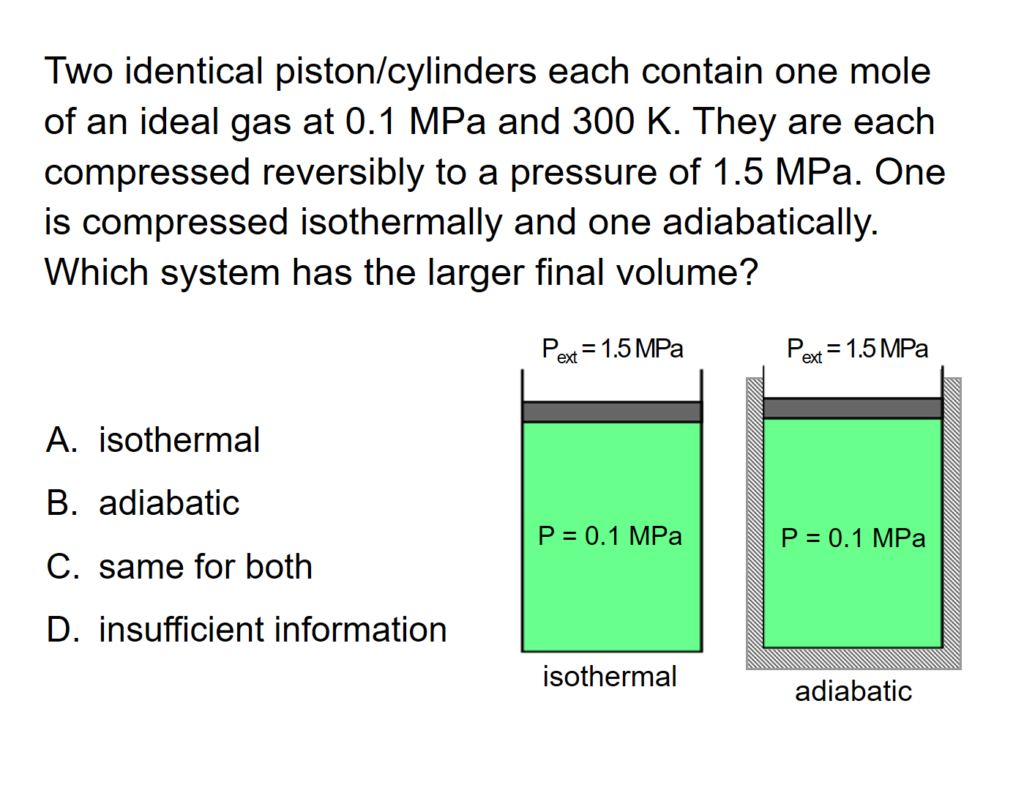#### Introduction to the First Law of Thermodynamics - Closed System: ConcepTest and Example Problem

Try to answer this ConcepTest and solve the example problem before using this module. Studies show that trying to answer the questions before studying material improves learning and retention. We suggest that you write down the reasons for your answers. By the end of this module, you should be able to answer these on your own. Answers will be given at the end of this module.##### Example Problem 1

Two containers at 350 K and 0.5-MPa pressure each contain 1.0 mol of an ideal gas.

$$C_P$$ = 31 J/(mol K)

Container A: constant pressure piston and cylinder

Container B: fixed volume

What is the temperature and pressure in each container after 8.5 kJ of heat are added to each?